Question

# Using the Hardy Weinberg Equilibrium equation, if you are given 25% are recessive, would the total...

Using the Hardy Weinberg Equilibrium equation, if you are given 25% are recessive, would the total amount of recessive alleles be 50% or something else, mostly wanting to know how to do this equation if anything but with real numbers. -Thanks

If 25% is given it is the frequency of the recessive genotype(q2 = 25/100 = 0.25) The frequency of recessive allele can be calculated as

q = √.25 = 0.5 p= 1- 0.5 = 0.5

Let us assume a population of 200 people in which p= 0.5 and q = 0.5

According to Hardy Weinberg equation, genotype frequency can be calculated from allele frequency by using the equation

p2 +q2 +2pq = 1

Where p2 = frequency of homozygous dominant AA = 0.5*0.5 = 0.25

Number of homozygous dominant individuals = 0.25 *200 = 50

q2 = frequency of homozygous recessive aa = 0.5 * 0.5 = 0.25

Number of homozygous recessive individuals = 0.25 * 200 = 50

2pq = frequency of heterozygous Aa= 2*0.5*0.5 = 0.5

Number of heterozygous individual = 0.5 *200 = 100

The allele frequency can be calculated by genotype frequency

p = (2*Number of homozygous dominant individuals + Number of heterozygous individuals)/2* total population

(2*50+100)/2*200 = 200/400 = 0.5

q = (2*Number of homozygous recessive individuals + Number of heterozygous individuals)/2* total population

(2*50+100)/2*200 = 200/400 = 0.5

p+q = 1

(Yes you can say 50% alleles are recessive in the population (0.5 * 100 = 50%), both dominant and recessive alleles have the same frequency in this case 0.5)

#### Earn Coins

Coins can be redeemed for fabulous gifts.

Similar Homework Help Questions
• ### 2.3 Problem 3 The Hardy-Weinberg equation is useful for predicting the percent of a hu- man...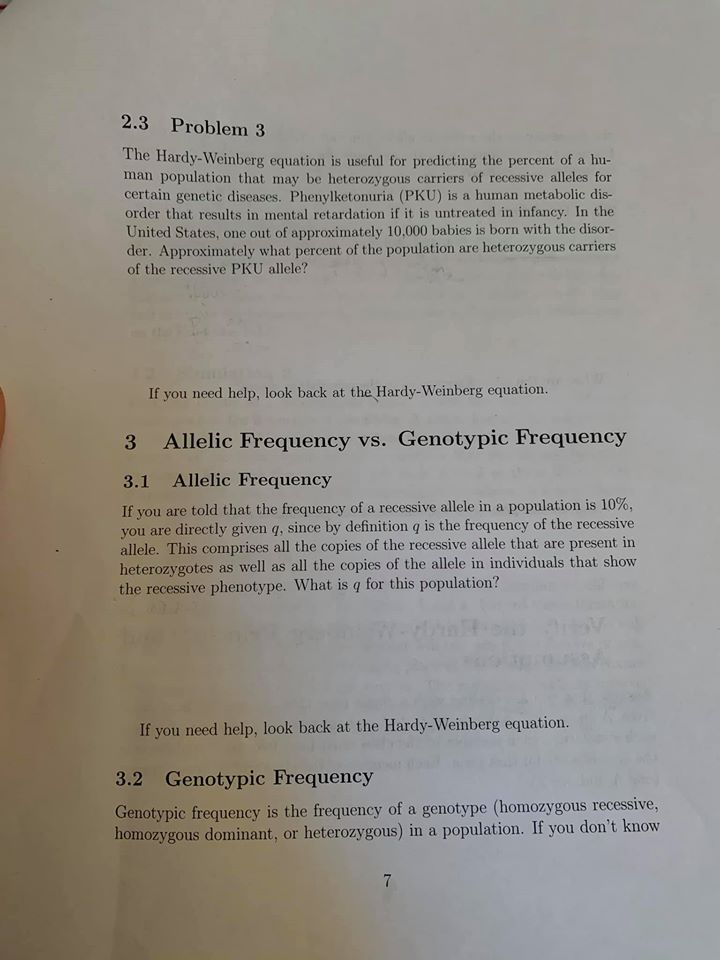2.3 Problem 3 The Hardy-Weinberg equation is useful for predicting the percent of a hu- man population that may be heterozygous carriers of recessive alleles for certain genetic diseases. Phenylketonuria (PKU) is a human metabolic dis- order that results in mental retardation if it is untreated in infancy. In the United States, one out of approximately 10.000 babies is born with the disor- der. Approximately what percent of the population are heterozygous carriers of the recessive PKU allele? If you...

• ### reting Data: Hardy-Weinberg Equation 2 of 10 you use the Hardy Weinberg equation to answer questions...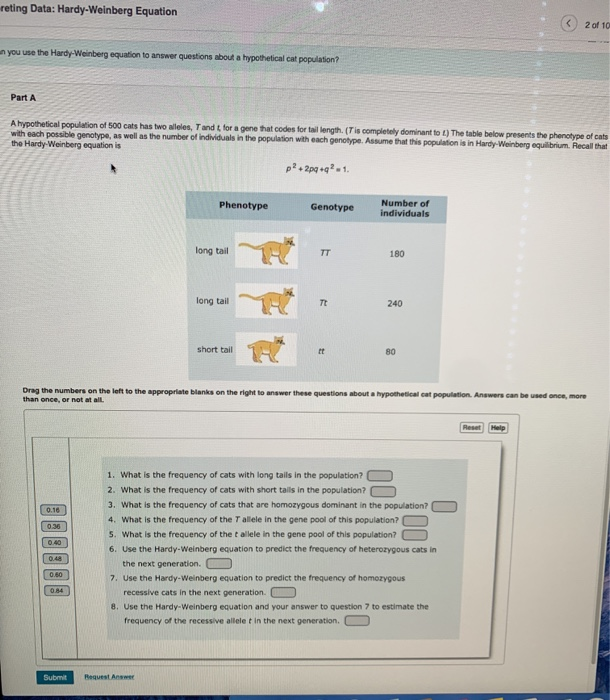reting Data: Hardy-Weinberg Equation 2 of 10 you use the Hardy Weinberg equation to answer questions about a hypotheticalcat population Part A A hypothetical population of 500 cats has two wees, Tandt for a gene that codes for tail length (Tis completely dominantot) The table below presents the phenotype of cats with each possible genotype, as well as the number of individuals in the population with each genotype. Assume that this population is in Hardy-Weinberg equilibrium Recall that the Hardy...

• ### 2. Hardy-Weinberg Equilibrium; chi-square test Sickle cell anemia is a recessive disorder caused by a recessive...

2. Hardy-Weinberg Equilibrium; chi-square test Sickle cell anemia is a recessive disorder caused by a recessive mutation (S) in the b-hemoglobin gene. 80% of affected SS individuals die before reproducing.   Heterozygotes (AS) and homozygous dominant (AA) individuals do not have sickle cell anemia. The table below shows the number of people of each genotype in a population of 100 people in population of Cameroon. Observed # individuals in a Cameroon population AA AS SS 62 32 6 What are the...

• ### EXERCISE 6 HARDY-WEINBERG EQUILIBRIUM Work in a small group or alone to complete this exercise. In...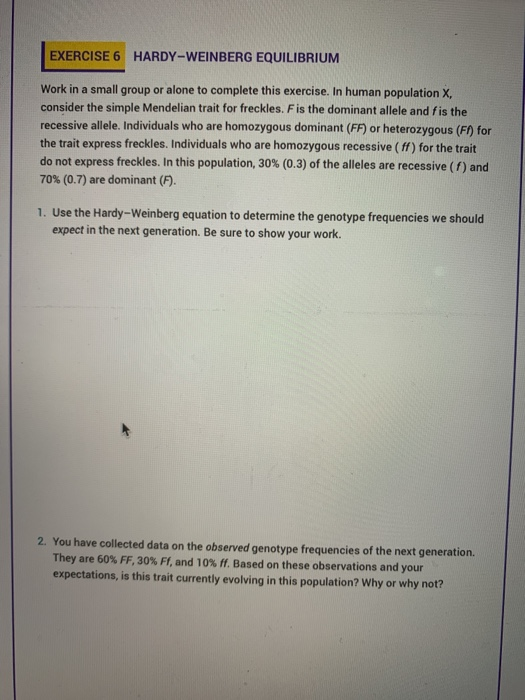EXERCISE 6 HARDY-WEINBERG EQUILIBRIUM Work in a small group or alone to complete this exercise. In human population X consider the simple Mendelian trait for freckles. F is the dominant allele and fis the recessive allele. Individuals who are homozygous dominant (FF) or heterozygous (F) for the trait express freckles. Individuals who are homozygous recessive (ff) for the trait do not express freckles. In this population, 30% (0.3) of the alleles are recessive (1) and 70% (0.7) are dominant (F)....

• ### In a population of wild sweet pea plants that you assume are in Hardy-Weinberg equilibrium, 1%...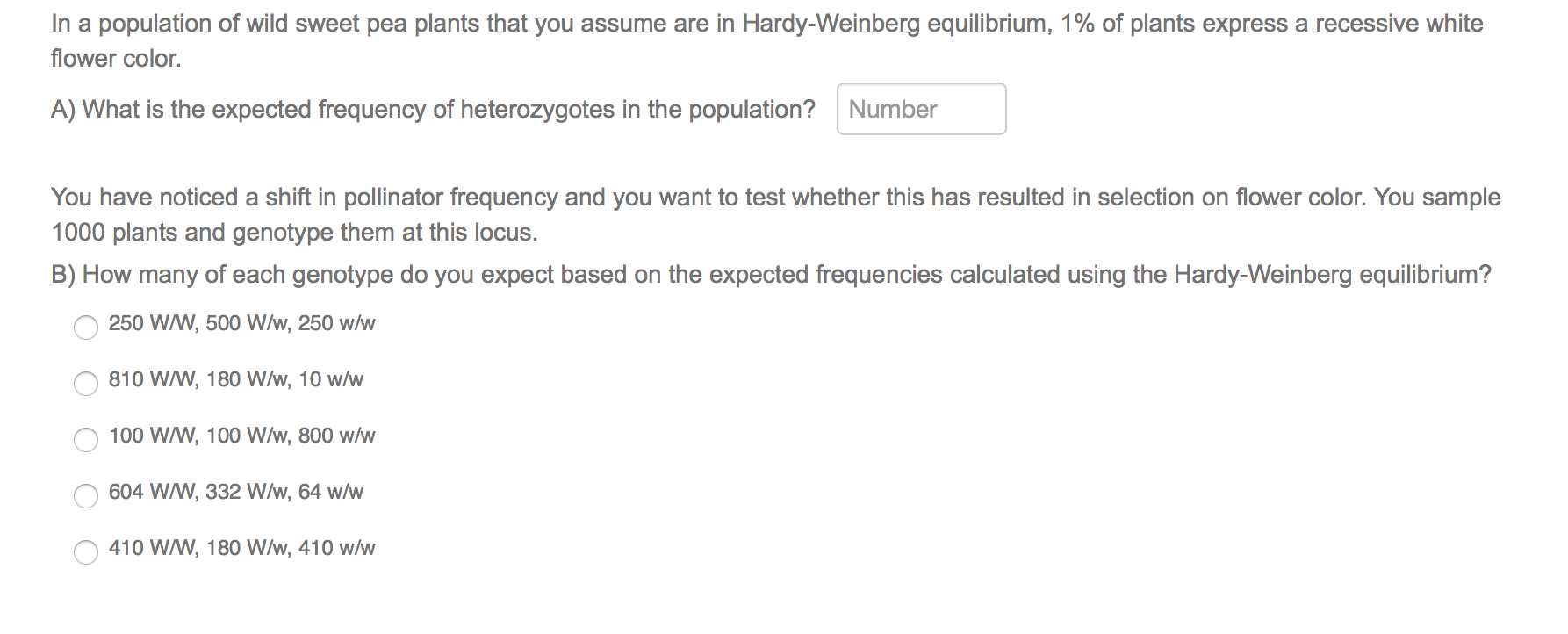In a population of wild sweet pea plants that you assume are in Hardy-Weinberg equilibrium, 1% of plants express a recessive white flower color. A) What is the expected frequency of heterozygotes in the population? Number You have noticed a shift in pollinator frequency and you want to test whether this has resulted in selection on flower color. You sample 1000 plants and genotype them at this locus. B) How many of each genotype do you expect based on the...

• ### If individuals homozygous for a recessive mutation make up 16% of a population at Hardy-Weinberg equilibrium,...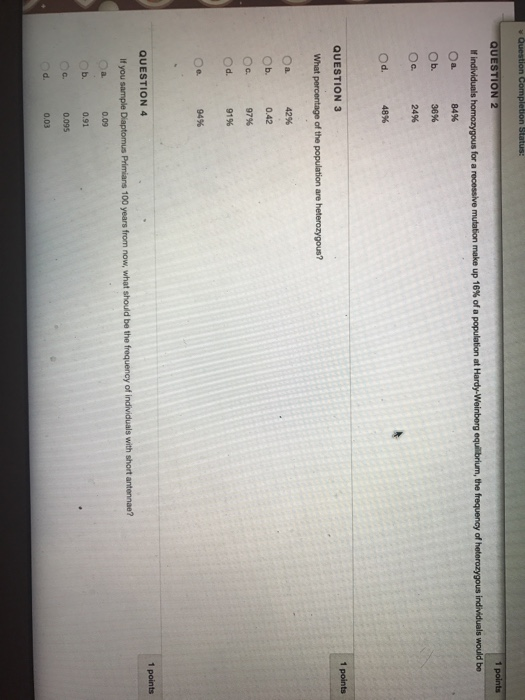If individuals homozygous for a recessive mutation make up 16% of a population at Hardy-Weinberg equilibrium, the frequency of heterozygous individuals would be a. 84% b. 36% c. 24% d. 48% What percentage of the population are heterozygous? a. 42% b. 0.42 c. 97% d. 91% e. 94% If you sample diatoms Parisians 100 years from now, what should be the frequency of individuals with short antennae? a. 0.09 b. 0.91 c. 0.095 d. 0.03

• ### You are a population geneticist studying Gene B in a population that is in Hardy-Weinberg equilibrium....

You are a population geneticist studying Gene B in a population that is in Hardy-Weinberg equilibrium. You sample a population to determine the genotypes at Gene B. You find that the freuency of homozygous recessive genotypes (bb) is 0.10. What would be the frequency of the dominant allele (B) in this population? A. 0.84 B. 0.4 C. 0.36 D. 0.6 E. 0.48

• ### 14. Describe how you can test the hypothesis of Hardy-Weinberg equilibrium if all you know is...

14. Describe how you can test the hypothesis of Hardy-Weinberg equilibrium if all you know is observed genotype frequencies. Use complete sentences.

• ### What is the Hardy Weinberg Equilibrium Model and how would one solve for the allelic frequency...

What is the Hardy Weinberg Equilibrium Model and how would one solve for the allelic frequency of the different genotypes based upon limited information. know what functional group makes DNA negatively charged and in what direction the DNA travels (towards the positive or the negative pole)!

• ### Which of the following statements regarding the Hardy-Weinberg Equation is TRUE? Answers: It can only be u...

Which of the following statements regarding the Hardy-Weinberg Equation is TRUE? Answers: It can only be used when allele and genotype frequencies remain constant across generations in a population It can only be used when allele and genotype frequencies change across generations in a population It has no assumptions It mathematically shows how recessive alleles in a population decrease over time It reveals when allele frequencies change over time in a population Assuming Hardy-Weinberg Equilibrium, what percentage of individuals in...